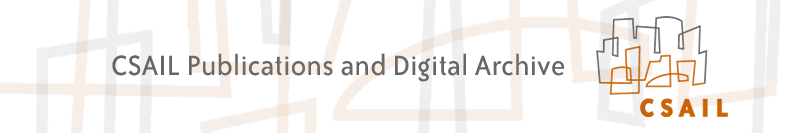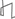Research Abstracts Home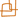CSAIL Digital Archive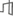Research Activities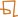CSAIL HomeResearch Abstracts - 2007IntroductionPerception & LearningPhysical, Biological & Social SystemsSystemsTheory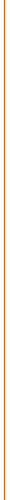### Generalized Hough Transforms For View-Independent Object Localization

#### Samuel I. Davies, Leslie P. Kaelbling & Tomas Lozano-Perez

##### Problem Definition

Given a 3D part-based model of an object, our goal is to create an efficient algorithm to find any view of the object in a 2D image. Existing methods accomplish this by learning a different 2D model for each viewpoint. Our approach generalizes pictorial structures  to work with 3D part-based models. The work also has roots in generalized hough transforms  and, more recently, the constellation model .

The object model consists of a set of point-like parts in 3D. The location of each point is drawn from a gaussian distribution about its mean with some variance.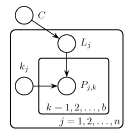n number of parts in the model (around 5 to 10) b number of candidate observations per part (each pixel may become a possible observation for a part, so b is potentially very large) C the object configuration (i.e. x, y, z, roll, pitch, yaw) Lj location of the jth part in the camera coordinate system (in 3D) kj selects one of the observations for the jth part as foreground, and the rest as background Pj,k the location of the kth candidate observation for the jth part on the image plane (in 2D)

The goal is to find the configuration C that most likely describes what we see in the image. For this best configuration, we also find the best assignment of observations (i.e. pixels on the screen) to parts in our model. In particular, we find maxC f(C):

 f(C) = log Pr(C|P) = log ∑k ∫LPr(C,L,k|P) dL ≈ log maxk ∫LPr(C,L,k|P) dL = log Pr(C) + ∑j=1...n maxk log ∫Lj Pr(Lj|C) Pr(kj) Pr(Pj,kj|kj,Lj) dLj

The integral in the last expression projects the 3D gaussian distribution associated with part j onto the 2D image (using perspective projection). This can be calculated by evaluating a line integral through the gaussian distribution along a ray starting from a pixel on the image (i.e. Pj,kj) and extending to infinity along all points in 3D space that could possibly project to that pixel.  We found that the closed form of this integral becomes simpler when we make the approximation of taking the integral along the line that goes through the camera's origin, including those points that lie behind the camera (i.e. we integrate along the whole line, not just a ray). This is a reasonable approximation if the gaussian lies a distance of several variances away from the camera's origin on the visible side, since the gaussian distribution drops quickly to zero.

##### The Algorithm

Looking at the form of f(C), we can see that it is a piecewise function whose pieces are defined by the boundaries of the maxk as it is summed for each part k. Each of n parts contributes as many as b pieces (one for each observation that could have been created by that part). As these pieces are summed, their regions are overlaid, breaking f(C) into as many as bn pieces. Since our goal is to find the object configuration C that maximizes f(C), this may mean evaluating the maximums of as many as bn pieces in f(C) to find the global maximum. (We are using the closed form for these functions to find the maximum in O(1) time, rather than iterative search techniques that don't have predictable running times.) Initially, this seems like an exponentially hard problem. Thankfully, it turns out that the some of the bn possible assignments of observations to parts are never expressed in the final form of f(C). For an example of this phenomenon, see the following figure: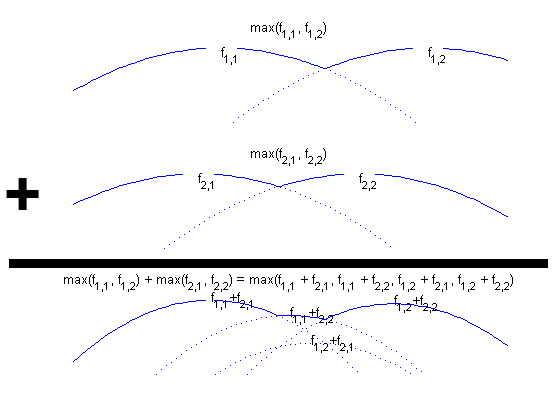Here you see that f1,2+f2,1 is never expressed in the lower graph, which is the sum of the upper two. Some preliminary experimental results suggest that this phenomenon may hide exponentially many of these hypothesis functions.

If there is a small number pieces in f(C), then there is only a small number of hypotheses that we need to examine. However, one question remains: in order to efficiently find the global maximum, how can we focus only on those hypotheses that are expressed in f(C) without examining all the exponential number of other hypotheses that are not expressed?

This is still an open question. We are looking at computational geometry techniques to address this question.

##### To Do

This is still preliminary research. If we do find a way to calculate the maximum of the objective function f(C) efficiently, there is still much work to do. In particular, we need to add

• an image model as in the pictorial structures model  so that each observation pixel and its surrounding region will be tested to see how good of a candidate it is.
• a provision in the model for parts that become hidden when the object rotates and occludes itself.
• a method to learn 3D object models from a set of training images.
##### References:

 Pedro F. Felzenszwalb and Daniel P. Huttenlocher. Pictorial Structures for Object Recognition. In Intl. Journal of Computer Vision, pp. 55--79, January 2005.

 D. H. Ballard. Generarlizing the Hough Transform to Detect Arbitrary Shapes. In Pattern Recognition. 13:111, 1981.

 R. Fergus, P. Perona, A. Zisserman. Object Class Recognition by Unsupervised Scale-Invariant Learning. In IEEE Conference on Computer Vision and Pattern Recognition (CVPR), 2003.

 Meg Aycinena. Unpublished work done in 2006.Computer Science and Artificial Intelligence Laboratory (CSAIL) The Stata Center, Building 32 - 32 Vassar Street - Cambridge, MA 02139 - USA tel:+1-617-253-0073 - publications@csail.mit.edu